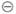Chapter 8.1, Problem 11ESMathematical Excursions (MindTap C...

4th Edition
Richard N. Aufmann + 3 others
ISBN: 9781305965584

Solutions

Chapter
SectionMathematical Excursions (MindTap C...

4th Edition
Richard N. Aufmann + 3 others
ISBN: 9781305965584
Textbook Problem

Evaluate each expression, where ⊕ and ⊖ indicate addition and subtraction, respectively, using a 12-hour clock.3 ⊖ 8

To determine

The subtraction 38 on a 12-hour clock.

Explanation

Given information: denote the subtraction on 12-hour.

Calculation:

38 denote the time 8 hours ago 3 o’clock...

Still sussing out bartleby?

Check out a sample textbook solution.

See a sample solution

The Solution to Your Study Problems

Bartleby provides explanations to thousands of textbook problems written by our experts, many with advanced degrees!

Get Started

Prove Formula 6 of Theorem 3.

Calculus (MindTap Course List)

Solve the equations in Exercises 112 for x (mentally, if possible). 7x+55=98

Finite Mathematics and Applied Calculus (MindTap Course List)